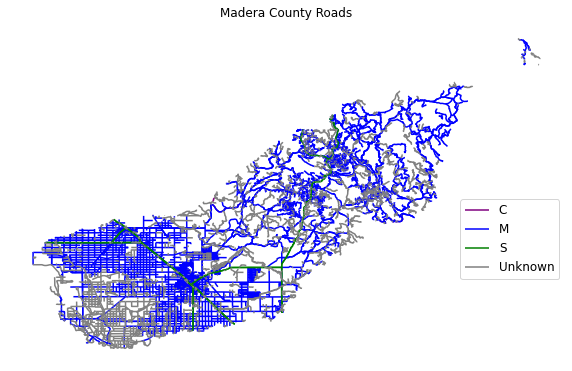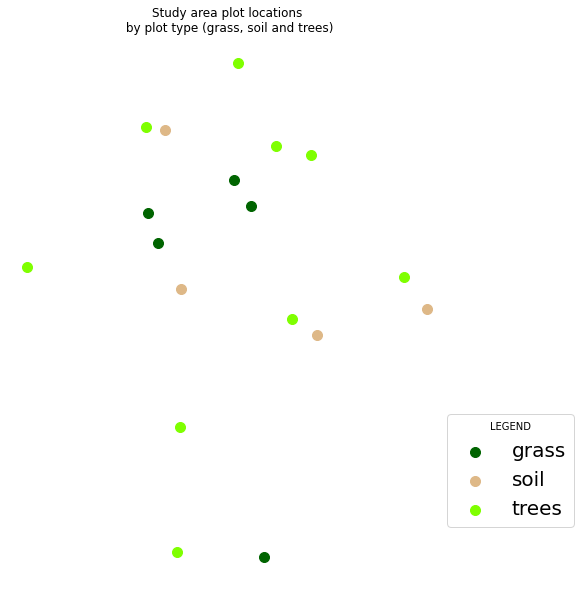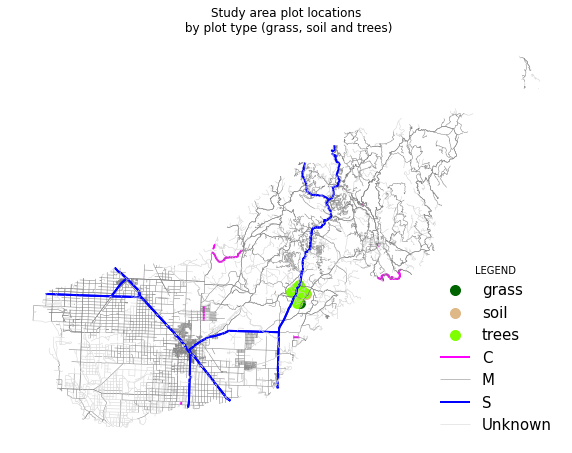# Lesson 2. Customize Map Legends and Colors in Python using Matplotlib: GIS in Python

## Learning Objectives

• Create a map containing multiple vector datasets, colored by unique attributes in Python.
• Add a custom legend to a map in Python with subheadings, unique colors.

## Create Custom Maps with Python

In this lesson, you will learn how to customize map symbology or the colors and symbols used to represent vector data in Python. There are many different ways to create maps in Python. In this lesson, you will use the geopandas and matplotlib.

To begin, import all of the required libraries.

# Import libraries
import os
import matplotlib.pyplot as plt
import numpy as np
from shapely.geometry import box
import geopandas as gpd
import earthpy as et

# Get the data & set working dir
data = et.data.get_data('spatial-vector-lidar')
os.chdir(os.path.join(et.io.HOME, 'earth-analytics', 'data'))

Downloading from https://ndownloader.figshare.com/files/12459464
Extracted output to /root/earth-analytics/data/spatial-vector-lidar/.


### Import Data

Next, import and explore your spatial data. In this case you are importing the same roads layer that you used in earlier lessons which is stored in shapefile (.shp) format.

# Import roads shapefile

# View data type

<class 'pandas.core.series.Series'>

# View unique attributes for each road in the data

['M' None 'S' 'C']


## Replace Missing Data Values

It looks like you have some missing values in your road types. You want to plot all road types even those that are set to None - which is python’s default missing data value. Change the roads with an RTTYP attribute of None to “unknown”.

# Map each value to a new value


['M' 'Unknown' 'S' 'C']


Data Tip: There are many different ways to deal with missing data in Python. Another way to replace all values of None is to use the .isnull() function like this: sjer_roads.loc[sjer_roads['RTTYP'].isnull(), 'RTTYP'] = 'Unknown'

If you plot your data using the standard geopandas .plot(), geopandas will select colors for your lines. You can add a legend using the legend=True argument however notice that the legend is composed of circles representing each line type rather than a line. You also don’t have full control over what color is applied to which line, line width and other symbology attributes.

fig, ax = plt.subplots(figsize=(14, 6))

categorical=True,
legend=True,
ax=ax)

leg = ax.get_legend()
leg.set_bbox_to_anchor((1.15,0.5))

ax.set_axis_off()

plt.show()


## Plot Color by Attribute

To plot a vector layer by attribute value so each road layer is colored according to it’s respective attribute value, and so the legend also represents that same symbology you need to do three things.

1. You need to create a dictionary that associates a particular color with a particular attribute value
2. You then need to loop through and apply that color to each attribute value
3. Finally you need to add a label argument to your plot so you can call ax.legend() to make your final legend.

To begin, create a dictionary that defines what color you want each road type to be plotting using.

# Create a dictionary where you assign each attribute value to a particular color
'S': 'green',
'C': 'purple',
'Unknown': 'grey'}

{'M': 'blue', 'S': 'green', 'C': 'purple', 'Unknown': 'grey'}


Next, you loop through each attribute value and plot the lines with that attribute value using the color specified in the dictionary. To ensure your legend generates properly, you add a label= argument to your plot call. The label value will be the attribute value that you used to plot. Below that value is defined by the ctype variable.

Then you can call ax.legend() to create a legend.

# Plot data
fig, ax = plt.subplots(figsize=(10, 10))

# Loop through each attribute type and plot it using the colors assigned in the dictionary

# Define the color for each group using the dictionary

# Plot each group using the color defined above
data.plot(color=color,
ax=ax,
label=ctype)

ax.legend(bbox_to_anchor=(1.0, .5), prop={'size': 12})

ax.set_axis_off()
plt.show()One way to customize plots is to create a dictionary that contains the symbology or colors and symbols that you wish to use for each attribute type in the map and legend.

You can adjust the width of your plot lines using the linewidth= attribute. If you set the linewidth to 4, you can create a truly ugly plot. In this example every line is width=4.

fig, ax = plt.subplots(figsize=(10, 10))

# Loop through each group (unique attribute value) in the roads layer and assign it a color
data.plot(color=color,
ax=ax,
label=ctype,
linewidth=4)  # Make all lines thicker

# Add title and legend to plot
ax.legend()
ax.set_axis_off()

plt.show()


### Adjust Line Width by Attribute

Similar to how you adjust colors, you can create a dictionary to adjust line widths. Then you can call dictionaryName[ctype] where dictionaryName is a dictionary of what line width you want to assign to each attribute value and ctype is the attribute value.

lineWidths = {'M': 1, 'S': 1, 'C': 4, 'Unknown': .5}

Here you are assigning the linewidth of each respective attibute value a line width as follows:

• M: 1
• S: 1
• C: 4
• Unknown = 4
# Create dictionary to map each attribute value to a line width
lineWidths = {'M': 1, 'S': 1, 'C': 4, 'Unknown': .5}

# Plot data adjusting the linewidth attribute
fig, ax = plt.subplots(figsize=(10, 10))
ax.set_axis_off()

data.plot(color=color,
ax=ax,
label=ctype,

# Assign each group to a line width using the dictionary created above
linewidth=lineWidths[ctype])

ax.legend()
ax.set(title='Madera County \n Line width varies by TYPE Attribute Value')
plt.show()


## Optional Challenge: Plot Line Width by Attribute

You can customize the width of each line, according to specific attribute value, too. To do this, you create a vector of line width values, and map that vector to the factor levels - using the same syntax that you used above for colors.

HINT: lwd=(vector of line width thicknesses)[spatialObject\$factorAttribute]

Create a plot of roads using the following line thicknesses:

1. unknown lwd = 3

2. M lwd = 1

3. S lwd = 2

4. C lwd = 1.5

## Customize Plot Legend

Above you created a legend using the label= argument and ax.legend(). You may want to move your legend around to make a cleaner map. You can use the loc= argument in the call to ax.legend() to adjust your legend location. This location can be numeric or descriptive.

Below you specify the loc= to be in the lower right hand part of the plot.

ax.legend(loc='lower right')

When you add a legend, you use the following elements to customize legend labels and colors:

• loc=(how-far-right, how-far-above-0): specify an x and Y location of the plot Or generally specify the location e.g. ‘bottom right’, ‘top’, ‘top right’, etc. If you use numeric values the first value is the position to the RIGHT of the plot and the second is the vertical position (how far above 0). Otherwise you can provide text for example “lower right” or “upper left”.
• fontsize: the size of the fonts used in the legend
• frameon: Boolean Values: True of False - if you want a box around your legend use True

The bbox_to_anchor=(1, 1) argument is also often helpful to customization the location further. Read more about that argument here in the matplotlib documentation.

lineWidths = {'M': 1, 'S': 2, 'C': 1.5, 'Unknown': 3}

fig, ax = plt.subplots(figsize=(10, 10))

# Loop through each attribute value and assign each
# with the correct color & width specified in the dictionary
label = ctype
data.plot(color=color,
ax=ax,
linewidth=lineWidths[ctype],
label=label)

ax.set(title='Madera County \n Line width varies by TYPE Attribute Value')

# Place legend in the lower right hand corner of the plot
ax.legend(loc='lower right',
fontsize=15,
frameon=True)

ax.set_axis_off()
plt.show()


See what happens when you set the frameon attribute of your legend to False and adjust the line widths - does your legend change? Also notice below the loc=() argument is given a tuple - two numbers that define the x and y location of the legend relative to the plot figure region.

lineWidths = {'M': 1, 'S': 2, 'C': 1.5, 'Unknown': 3}

fig, ax = plt.subplots(figsize=(10, 10))

label = ctype
data.plot(color=color,
ax=ax,
linewidth=lineWidths[ctype],
label=label)

ax.set(title='Madera County \n Line width varies by TYPE Attribute Value')
ax.legend(loc=(1, .5),
fontsize=15,
frameon=False,
title="LEGEND")

ax.set_axis_off()
plt.show()


Now, adjust both the plot colors and line widths.

roadPalette = {'M': 'grey', 'S': "blue",
'C': "magenta", 'Unknown': "lightgrey"}

lineWidths = {'M': 1, 'S': 2, 'C': 1.5, 'Unknown': 3}

fig, ax = plt.subplots(figsize=(10, 10))

label = ctype

data.plot(color=color,
ax=ax,
linewidth=lineWidths[ctype],
label=label)

ax.legend(loc='lower right',
fontsize=20,
frameon=False)

ax.set_axis_off()

plt.show()


## Plot Lines by Attribute

Play with colors one more time. Create a plot that emphasizes only roads designated as C or S (County or State). To emphasize these types of roads, make the lines that are assigned the RTTYP attribute of C or S, THICKER than the other lines.

Be sure to add a title and legend to your map! You might consider a color palette that has all County and State roads displayed in a bright color. All other lines can be grey.

# Define colors and line widths
roadPalette = {'M': 'grey', 'S': "blue",
'C': "magenta", 'Unknown': "lightgrey"}

lineWidths = {'M': .5, 'S': 2, 'C': 2, 'Unknown': .5}

fig, ax = plt.subplots(figsize=(10, 10))

label = ctype

data.plot(color=color,
ax=ax,
linewidth=lineWidths[ctype],
label=label)

ax.legend(loc='lower right',
fontsize=20,
frameon=False)

ax.set_axis_off()
plt.show()


Next, add another layer to your map to see how you can create a more complex map with a legend that represents both layers. You will add the same SJER_plot_centroids shapefile that you worked with in previous lessons to your map.

If you recall, this layer contains 3 plot_types: grass, soil and trees.

# Import points layer
sjer_plots_path = os.path.join("spatial-vector-lidar",
"california",
"neon-sjer-site",
"vector_data",
"SJER_plot_centroids.shp")

# View first 5 rows

Plot_IDPointnorthingeastingplot_typegeometry
0SJER1068center4111567.818255852.376treesPOINT (255852.376 4111567.818)
1SJER112center4111298.971257406.967treesPOINT (257406.967 4111298.971)
2SJER116center4110819.876256838.760grassPOINT (256838.760 4110819.876)
3SJER117center4108752.026256176.947treesPOINT (256176.947 4108752.026)
4SJER120center4110476.079255968.372grassPOINT (255968.372 4110476.079)

Just like you did above, create a dictionary that specifies the colors associated with each plot type. Then you can plot your data just like you did with the lines

pointsPalette = {'trees': 'chartreuse',
'grass': 'darkgreen', 'soil': 'burlywood'}

lineWidths = {'M': .5, 'S': 2, 'C': 2, 'Unknown': .5}

fig, ax = plt.subplots(figsize=(10, 10))

for ctype, data in sjer_plots.groupby('plot_type'):
color = pointsPalette[ctype]
label = ctype
data.plot(color=color,
ax=ax,
label=label,
markersize=100)

ax.set(title='Study area plot locations\n by plot type (grass, soil and trees)')

ax.legend(fontsize=20,
frameon=True,
loc=(1, .1),
title="LEGEND")

ax.set_axis_off()
plt.show()A similar approach can be taken to customize points on a plot.

### Overlay points on top of roads

Next, plot the plot data on top of the roads layer. Then create a custom legend that contains both lines and points.

NOTE: In this example, the projection for the roads layer has to match the projection of the SJER plot locations.

# Reproject the data

fig, ax = plt.subplots(figsize=(10, 10))

for ctype, data in sjer_plots.groupby('plot_type'):
color = pointsPalette[ctype]
label = ctype
data.plot(color=color,
ax=ax,
label=label,
markersize=100)

label = ctype
data.plot(color=color,
ax=ax,
linewidth=lineWidths[ctype],
label=label)

ax.set(title='Study area plot locations\n by plot type (grass, soil and trees)')

ax.legend(fontsize=15,
frameon=False,
loc=('lower right'),
title="LEGEND")

ax.set_axis_off()
plt.show()You can customize points and lines in one plot by using the ax object for both plots.